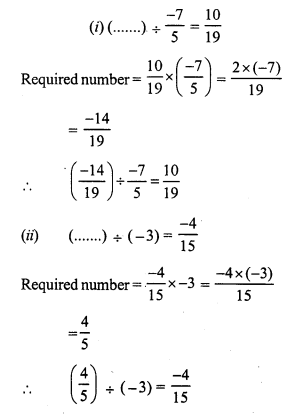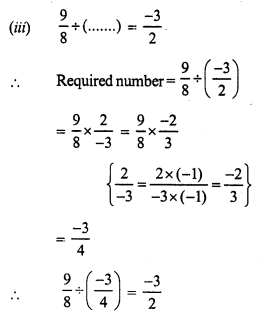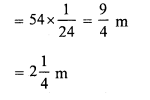# RS Aggarwal Class 7 Solutions Chapter 4 Rational Numbers Ex 4F

In this chapter, we provide RS Aggarwal Solutions for Class 7 Chapter 4 Rational Numbers Ex 4F for English medium students, Which will very helpful for every student in their exams. Students can download the latest RS Aggarwal Solutions for Class 7 Chapter 4 Rational Numbers Ex 4F Maths pdf, free RS Aggarwal Solutions Class 7 Chapter 4 Rational Numbers Ex 4F Maths book pdf download. Now you will get step by step solution to each question.

### RS Aggarwal Solutions for Class 7 Chapter 4 Rational Numbers Ex 4F Download PDF

Question 1.
Solution:(vii) Reciprocal of -1 = -1
(viii) Reciprocal of 0 does not exist.

Question 2.
Solution:Question 3.
Solution:Question 4.
Solution:Question 5.
Solution:Question 6.
Solution:Question 7.
Solution:
Product of two number = 10
One number = -8
Second number = 10 ÷ (-8)Question 8.
Solution:
Product of two rational numbers = – 9
One number = -12
Second number = (-9) ÷ (-12)Question 9.
Solution:Question 10.
Solution:Question 11.
Solution:
Cloth required for 24 pairs of trousers =54 m
Cloth required for one pair = (54 ÷ 24) mQuestion 12.
Solution:
Total length of rape = 30 mQuestion 13.
Solution:All Chapter RS Aggarwal Solutions For Class 7 Maths

—————————————————————————–

All Subject NCERT Exemplar Problems Solutions For Class 7

All Subject NCERT Solutions For Class 7

*************************************************

I think you got complete solutions for this chapter. If You have any queries regarding this chapter, please comment on the below section our subject teacher will answer you. We tried our best to give complete solutions so you got good marks in your exam.

If these solutions have helped you, you can also share rsaggarwalsolutions.in to your friends.